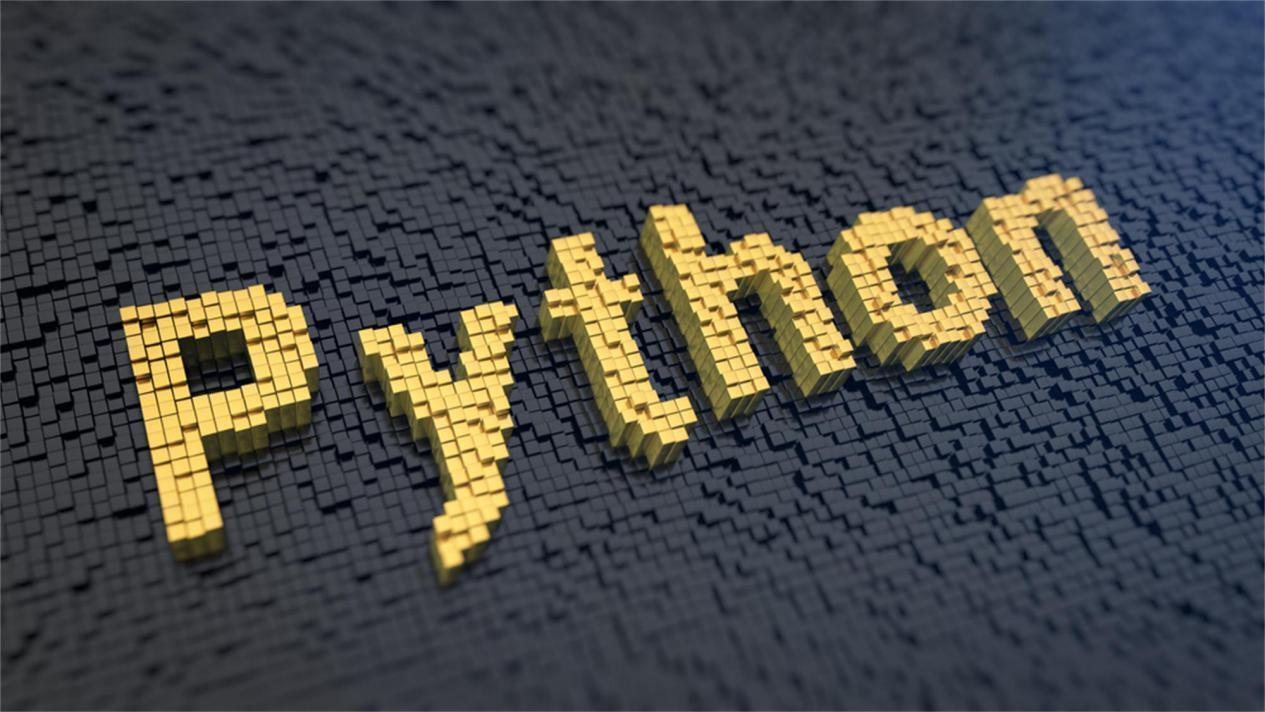Python 正则表达式正则表达式（RegularExpression, re）

• 是一个计算机科学的概念

• 用于使用单个字符串来描述，匹配符合某个规则的字符串

• 常常用来检索，替换某些模式的文本

正则的写法

• .(点号): 表示任意一个字符，除了\n，比如查找所有的一个字符 .

• []: 匹配中括号中列举的任意字符，比如[L,Y,0], LLY, Y0, LIU

• \d: 任意一个数字

• \D: 除了数字都可以

• \s: 表示空格，tab 键

• \S: 除了空白符号

• \w: 单词字符，就是 a-z, A-Z, 0-9, _

• \W: 除了“\w”表示的都可以

• : 表示前面内容重复零次或者多次, \w

• +: 表示前面内容至少出现一次

• ?: 前面才出现的内容零次或者一次

• {m,n}: 允许前面内容出现最少 m 次，最多 n 次

• ^: 匹配字符串的开始

• \$: 匹配字符串的结尾

• \b: 匹配单词的边界

• (): 对正则表达式内容进行分组，从第一个括号开始，编号逐渐增大

• \A: 只匹配字符串开头，\Aabcd, 则 abcd

• \Z: 只匹配字符串末尾，abcd\Z, abcd

• |: 左右任意一个

• (?P<name>...): 分组，除了原来的编号再制定一个别名，(?P<id>12345){2}, 1234512345

• (?P=name): 引用分组

RE 使用大致步骤

1. 使用 compile 将表示正则的字符串编译为一个 pattern 对象

2. 通过 pattern 对象提供一系列方法度文本进行查找匹配，获得匹配结果，一个 Match 对象

3. 最后使用 Match 对象提供的属性和方法获得信息，根据需要进行操作

RE 常用函数

• group(): 获得一个或者多个分组匹配的字符串，当要获得整个匹配的子串时，直接使用 group 或者 group(0)

• start: 获取分组匹配的子串在整个字符串中的起始位置，参数默认 0

• end: 获取分组匹配的子串在整个字符串中的结束位置，默认为 0

• span: 返回的结构技术(start(group), end(group))

# 导入相关包import re
# 查找数字# r表示字符串不转义p = re.compile(r'\d+')# 在字符串“one12twothree33456four78”中进行查找，按照规则p制定的正则进行查找# 返回结果是None表示没有找到，否则会返回match对象m = p.match("one12twothree33456four78")
print(m)

None

# 导入相关包import re
# 查找数字# r表示字符串不转义p = re.compile(r'\d+')# 在字符串“one12twothree33456four78”中进行查找，按照规则p制定的正则进行查找# 返回结果是None表示没有找到，否则会返回match对象# 参数3,6表示在字符串中查找的范围m = p.match("one12twothree33456four78", 3, 26)
print(m)
# 上述代码说明的问题# 1. match可以输入参数表示起始位置# 2. 查找到的结果只包含一个，表示第一次进行匹配成功的内容

<_sre.SRE_Match object; span=(3, 5), match='12'>

print(m)print(m.start(0))print(m.end(0))

1235

import re# I 表示忽略掉大小写p = re.compile(r'([a-z]+) ([a-z]+)', re.I)
m = p.match("I am really love you")print(m)

<_sre.SRE_Match object; span=(0, 4), match='I am'>

print(m.group(0))print(m.start(0))print(m.end(0))

I am04

print(m.group(1))print(m.start(1))print(m.end(1))

I01

print(m.group(2))print(m.start(2))print(m.end(2))

am24

print(m.groups())

('I', 'am')

查找

• search(str, [, pos[, endpos]]): 在字符串中查找匹配，pos 和 endpos 表示起始位置

• findall: 查找所有

• finditer：查找，返回一个 iter 结果

import re
p = re.compile(r'\d+')
m = p.search("one12two34three567four")
print(m.group())

12

rst = p.findall("one12two34three567four")print(type(rst))
print(rst)

<class 'list'>['12', '34', '567']

sub 替换

• sub(rep1, str[, count])

# sub替换案例import re
# \w 包含数字和英文字母p = re.compile(r'(\w+) (\w+)')
s = "hello 123 wang 456, i love you"
rst = p.sub(r'Hello world', s)print(rst)

Hello world Hello world, Hello world you

匹配中文

• 大部分中文表示范围是[u4e00-u9fa5], 不包括全角标点

import re
title = '世界 你好， hello moto'
p = re.compile(r'[\u4e00-\u9fa5]+')rst = p.findall(title)
print(rst)

['世界', '你好']

贪婪和非贪婪

• 贪婪：尽可能多的匹配, (*)表示贪婪匹配

• 非贪婪：找到符合条件的最小内容即可，(?)表示非贪婪

• 正则默认使用贪婪匹配

import re
title = u'<div>name</div><div>age</div>'
p1 = re.compile(r'<div>.*</div>')p2 = re.compile(r'<div>.*?</div>')
m1 = p1.search(title)print(m1.group())
m2 = p2.search(title)print(m2.group())

<div>name</div><div>age</div><div>name</div>评论